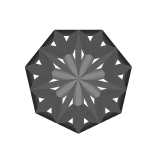3:28 AM
0I note this question is going to close by the Mathematic question(not a Mathematica). I think it is a function of Mathematica to try or practice with MMA when learning Mathematic. We can't simply categorize this need as a purely mathematical problem and then ask to close it. In this post, we can ...